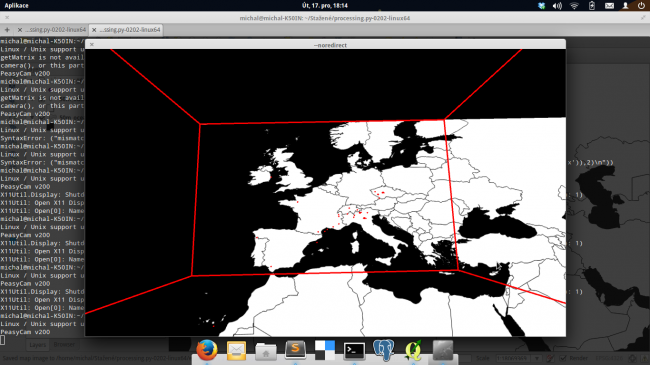#### Howdy, Stranger!

We are about to switch to a new forum software. Until then we have removed the registration on this forum.

# How to map GPS coordinates to processing canvas coordinates?

edited December 2013

Hello, I have a list of GPS coordinates that I would like to show on a map in processing. From reading the docs I understood that map() function might be handy for translating GPS coordinates to the canvas. However, I can't make it work. I tried this:

``````x = map(lon, -37.66195, 85.73613, 0, width)
y = map(lat, 23.76628, 65.81310, 0, height)
``````

where the numbers are most left/most right, most bottom/most up bounds of a displayed area. Draw() function looks like this:

``````def draw():
global basemap
global tweets
rotateX(-0.5)
background(0)
noFill()
stroke(255,0,0)
box(600,600,424)
translate(-300,300,-212)
rotateX(HALF_PI)
image(basemap,0,0)
for i in range(0, len(tweets)):
strokeWeight(3)
point(tweets[i].get('x'), tweets[i].get('y'),2)
``````

I am currently stuck and don't know how to solve this problem, so any help would be really appreciated. UPDATE: whole code

``````#!/usr/bin/python
# -*- coding: utf-8 -*-

from peasy import PeasyCam
import json

basemap = None
tweets = []

def setup():
global basemap
global tweets
count = data.size()
for i in range(0, count):
lon = data.getJSONObject(i).getJSONObject('coordinates').getJSONArray('coordinates').getFloat(0)
lat = data.getJSONObject(i).getJSONObject('coordinates').getJSONArray('coordinates').getFloat(1)
x = map(lon, -37.66195, 85.73613, 300, width)
y = map(lat, 23.76628, 65.81310, 0, height)
tweets.append({'x': x, 'y': y})

size(600, 600, P3D)
cam = PeasyCam(this,0,-200,200,700)
cam.setMinimumDistance(10)
cam.setMaximumDistance(1000)
cam.rotateX(0.5);

def draw():
global basemap
global tweets
rotateX(-0.5)
background(0)
noFill()
stroke(255,0,0)
box(600,600,424)

translate(-300,300,-212)
rotateX(HALF_PI)
image(basemap,0,0)

for i in range(0, len(tweets)):
strokeWeight(3)
point(tweets[i].get('x'), tweets[i].get('y'),2)
``````
Tagged:

• Your code seems like Python to me! Either you use Processing's Python (or even Ruby) version or adapt that for default Java!

That line #12 would be kinda like below in Java:

``````for (Tweet t: tweets)   point(t.get("x"), t.get("y"), 2);
``````
• You are right, I am using Python. But that should not be causing the problem I suppose. UPDATE: To be precise, the points are displayed, but not in right position. Might using P3D environment be causing this problem?

• How do you know they are not in the right position? Do you have some background map? If so, you need to check in which projection that map is.

However, if your background map uses Mercator projection, you need to use a different conversion (e.g. by using my helper class).

• Hello tnagel, you are right, I am using background map in WGS84 (which is an equirectangular projection). That is how I can tell the points are far off the right position. I updated the question with the complete source code. I got the bounds from QGIS using coordinates of the current extent.

• edited December 2013

Can you post your map image (basemap_wgs.jpg)? I assume that actually is in Mercator. (WGS84 is not a projection, and only is distortion free when used on a sphere.)

• can you explain what these two lines are for, specifically the numbers...

``````    x = map(lon, -37.66195, 85.73613, 300, width)
y = map(lat, 23.76628, 65.81310, 0, height)
``````

i've a feeling you're setting the range to match the range of your data BUT it needs to be the full possible range or the mapping won't work. ie LON still needs to use -180 to 180 and LAT needs to be -90 to 90 (watch the y axis though - (0,0) is top left, (0, height) is bottom left). but this is an assumption 8)

• @tnagel: WGS84 is both an ellipsoid and a CRS. The map is in WGS84.

@koogs: I am only displaying data in between those values, so I do not need the entire span of -180 to 180 degrees. The map also covers just the area defined by boundaries.

I got already to this which seems to be almost perfect except for the small shift (e. g. the point south of Catalania should not be in the sea).• Are you using PeasyCam? Maybe this is causing the small shifts... are the points and the map on the same Z value?

• @colouredmirrorball: Yes I am and yes, they are on the same Z value. Dunno why this happens. The original data fits perfectly on the map (tested in QGIS).

• Strange. The last line: `point(tweets[i].get('x'), tweets[i].get('y'),2)`, doesn't this indicate the points are two pixels above the map? But two pixels is small... that shouldn't cause such large deviations.

• @colouredmirrorball: yeah it does, but as you said, it does not really matters. However, I think it might be caused by getting coordinates of extents from qgis: it seems to be rounding them up to integers. I'll try to get them as precise floats, hope this will help.

I was thinking about that as well but couldn't find where they could get transformed to ints in your code. It's a good idea to do a println() on the data so you can check if they are integers.

• i did not read the thread very well, will do it tomorrow...but converting coordinates to pixels can be tricky some times... i use something like this

``````    public Vec2D geoToPixel(Vec2D v, int AppletWidth, int AppletHeight){
return new Vec2D(AppletWidth * (v.x - this.left)
/ (this.right - this.left), AppletHeight - AppletHeight
* (v.y - this.bottom) / (this.top- this.bottom));
}
``````

i just copied/paste this...the this.bottom etc are the boundaries of the pic/map that you use